Скачать презентацию Chapter 11 Probability Copyright 2015 2011 and

ac775d8bc5b60f9c9f5845ea4efc2592.ppt

• Количество слайдов: 24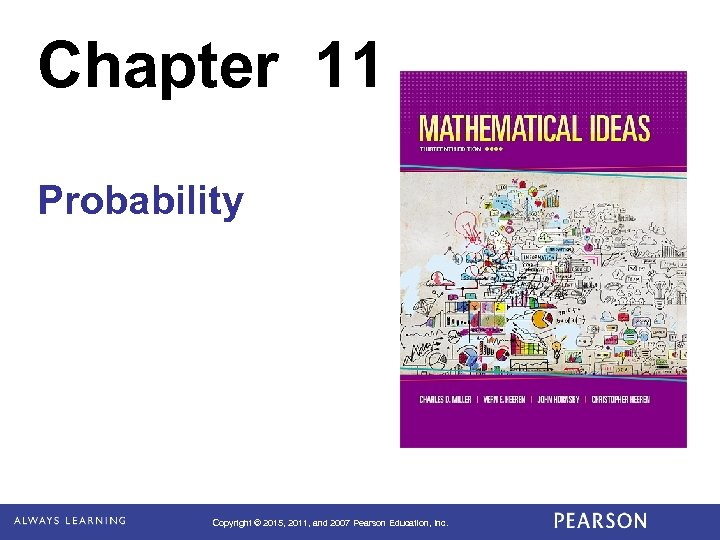Chapter 11 Probability Copyright © 2015, 2011, and 2007 Pearson Education, Inc.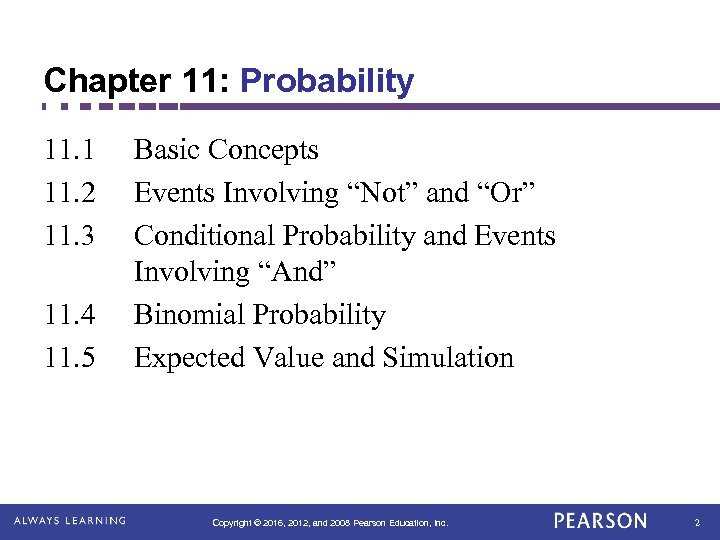Chapter 11: Probability 11. 1 11. 2 11. 3 11. 4 11. 5 Basic Concepts Events Involving “Not” and “Or” Conditional Probability and Events Involving “And” Binomial Probability Expected Value and Simulation Copyright © 2016, 2012, and 2008 Pearson Education, Inc. 2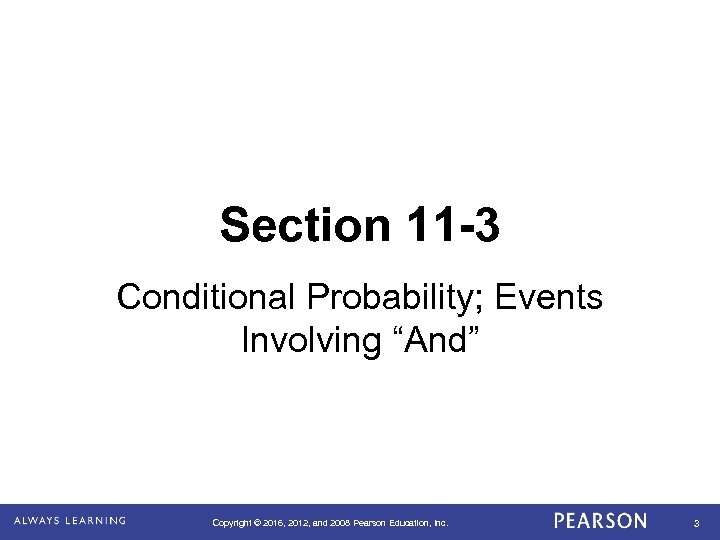Section 11 -3 Conditional Probability; Events Involving “And” Copyright © 2016, 2012, and 2008 Pearson Education, Inc. 3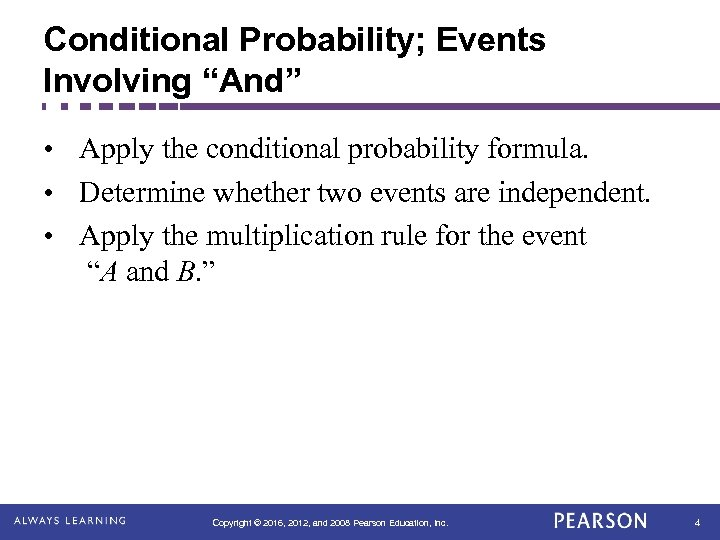Conditional Probability; Events Involving “And” • Apply the conditional probability formula. • Determine whether two events are independent. • Apply the multiplication rule for the event “A and B. ” Copyright © 2016, 2012, and 2008 Pearson Education, Inc. 4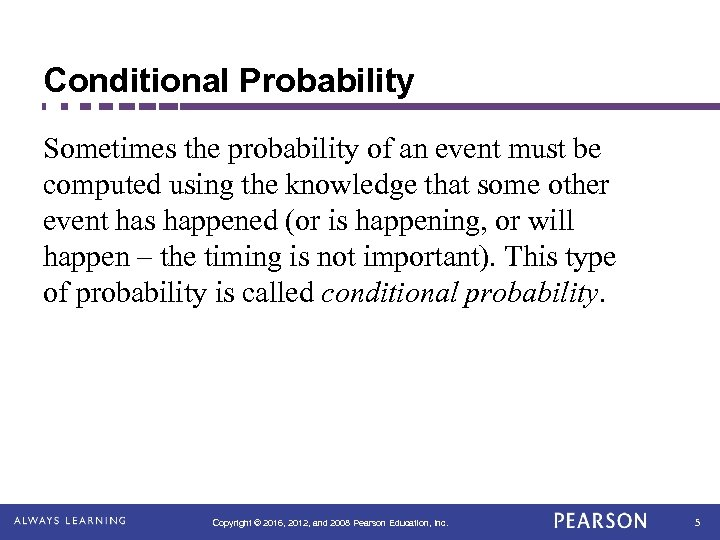Conditional Probability Sometimes the probability of an event must be computed using the knowledge that some other event has happened (or is happening, or will happen – the timing is not important). This type of probability is called conditional probability. Copyright © 2016, 2012, and 2008 Pearson Education, Inc. 5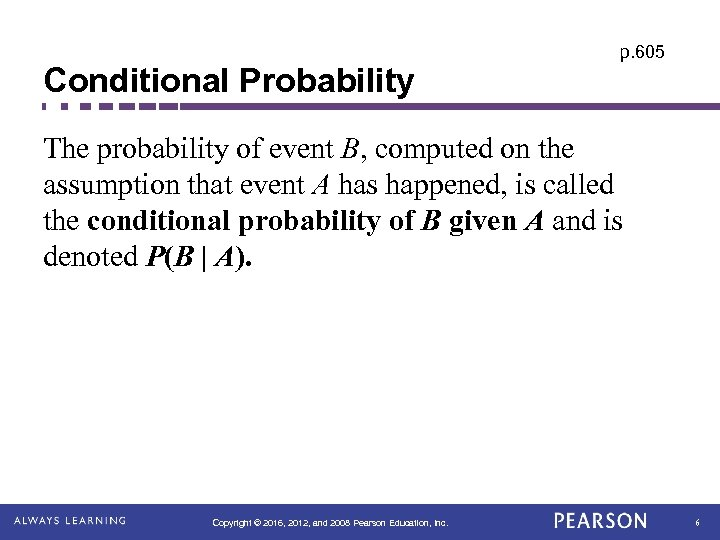Conditional Probability p. 605 The probability of event B, computed on the assumption that event A has happened, is called the conditional probability of B given A and is denoted P(B | A). Copyright © 2016, 2012, and 2008 Pearson Education, Inc. 6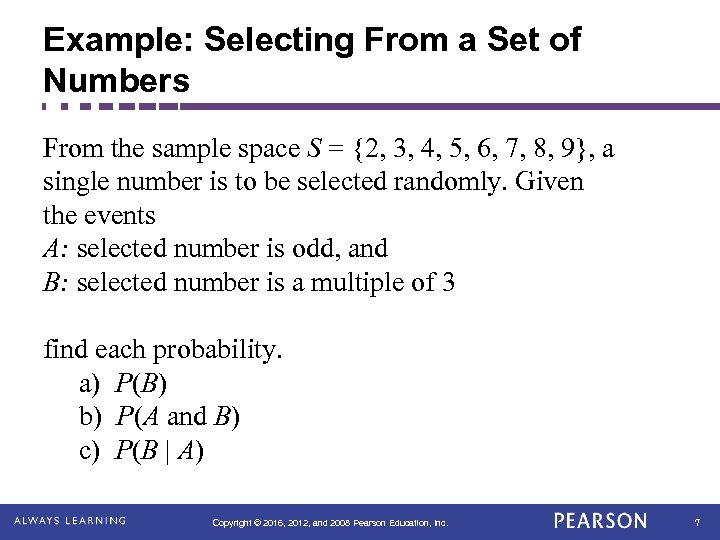Example: Selecting From a Set of Numbers From the sample space S = {2, 3, 4, 5, 6, 7, 8, 9}, a single number is to be selected randomly. Given the events A: selected number is odd, and B: selected number is a multiple of 3 find each probability. a) P(B) b) P(A and B) c) P(B | A) Copyright © 2016, 2012, and 2008 Pearson Education, Inc. 7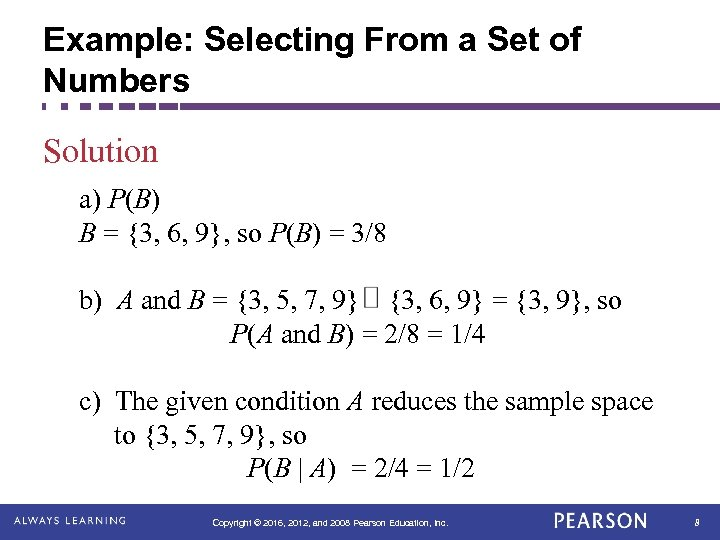Example: Selecting From a Set of Numbers Solution a) P(B) B = {3, 6, 9}, so P(B) = 3/8 b) A and B = {3, 5, 7, 9} {3, 6, 9} = {3, 9}, so P(A and B) = 2/8 = 1/4 c) The given condition A reduces the sample space to {3, 5, 7, 9}, so P(B | A) = 2/4 = 1/2 Copyright © 2016, 2012, and 2008 Pearson Education, Inc. 8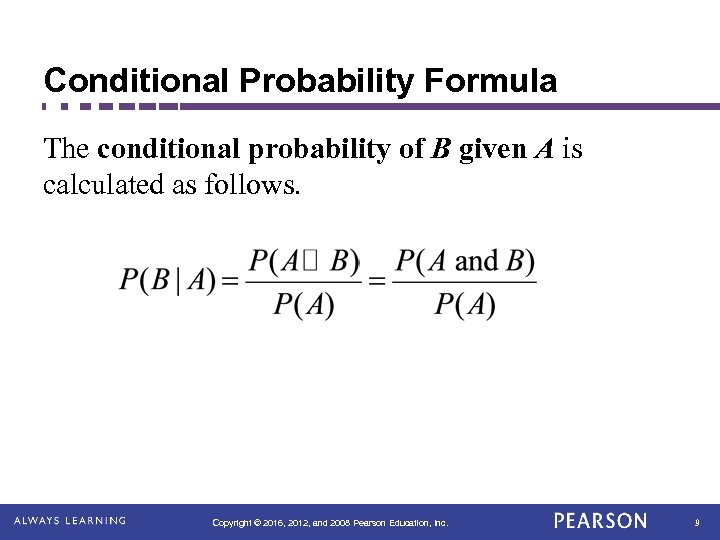Conditional Probability Formula The conditional probability of B given A is calculated as follows. Copyright © 2016, 2012, and 2008 Pearson Education, Inc. 9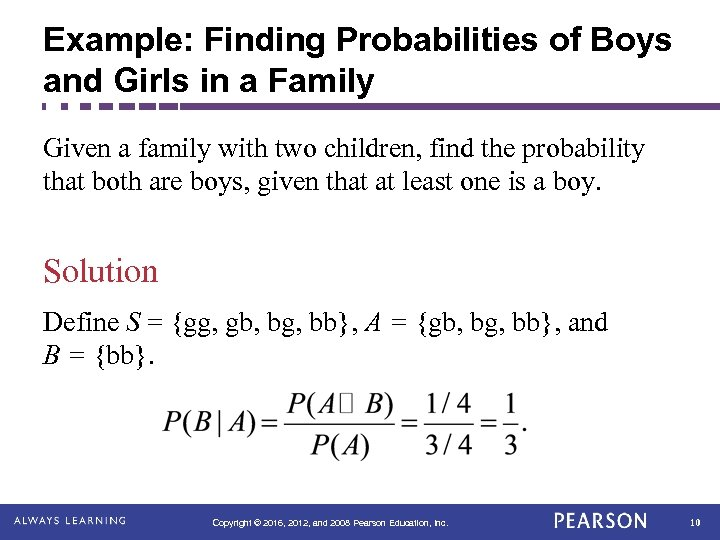Example: Finding Probabilities of Boys and Girls in a Family Given a family with two children, find the probability that both are boys, given that at least one is a boy. Solution Define S = {gg, gb, bg, bb}, A = {gb, bg, bb}, and B = {bb}. Copyright © 2016, 2012, and 2008 Pearson Education, Inc. 10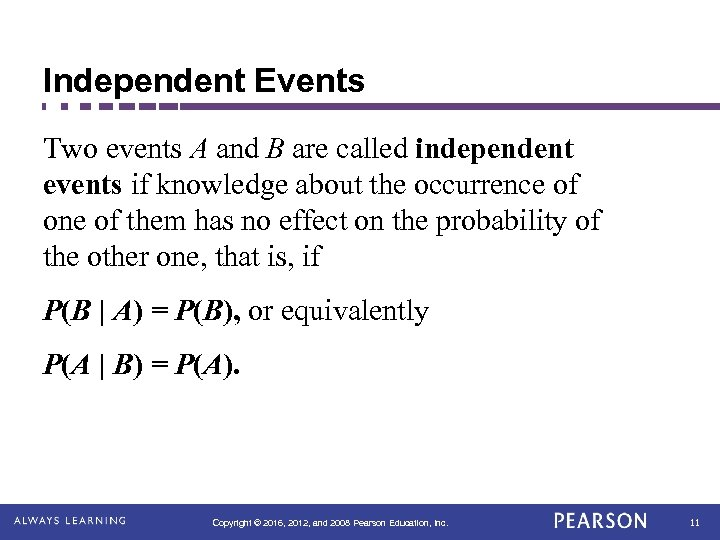Independent Events Two events A and B are called independent events if knowledge about the occurrence of one of them has no effect on the probability of the other one, that is, if P(B | A) = P(B), or equivalently P(A | B) = P(A). Copyright © 2016, 2012, and 2008 Pearson Education, Inc. 11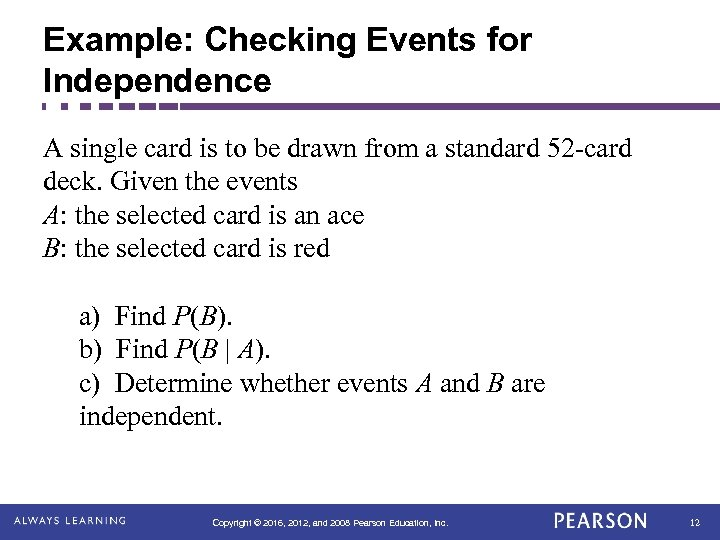Example: Checking Events for Independence A single card is to be drawn from a standard 52 -card deck. Given the events A: the selected card is an ace B: the selected card is red a) Find P(B). b) Find P(B | A). c) Determine whether events A and B are independent. Copyright © 2016, 2012, and 2008 Pearson Education, Inc. 12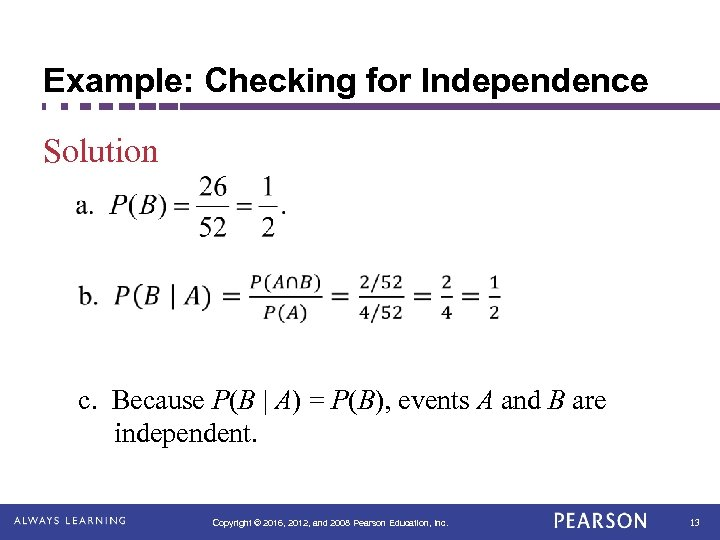Example: Checking for Independence Solution c. Because P(B | A) = P(B), events A and B are independent. Copyright © 2016, 2012, and 2008 Pearson Education, Inc. 13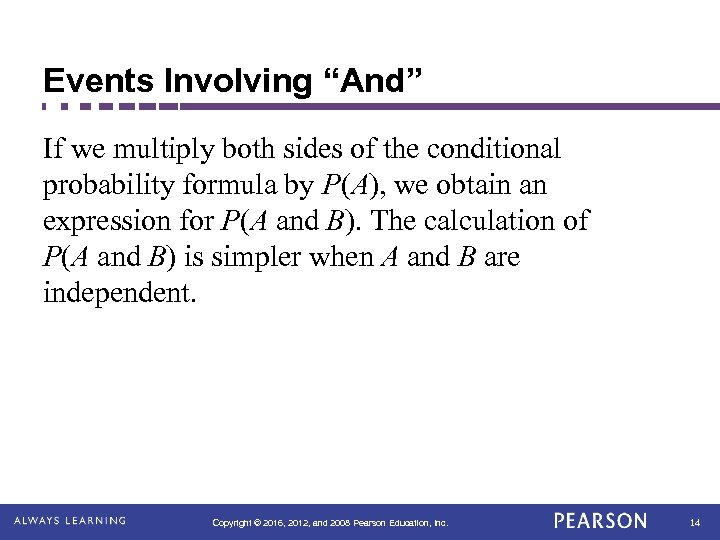Events Involving “And” If we multiply both sides of the conditional probability formula by P(A), we obtain an expression for P(A and B). The calculation of P(A and B) is simpler when A and B are independent. Copyright © 2016, 2012, and 2008 Pearson Education, Inc. 14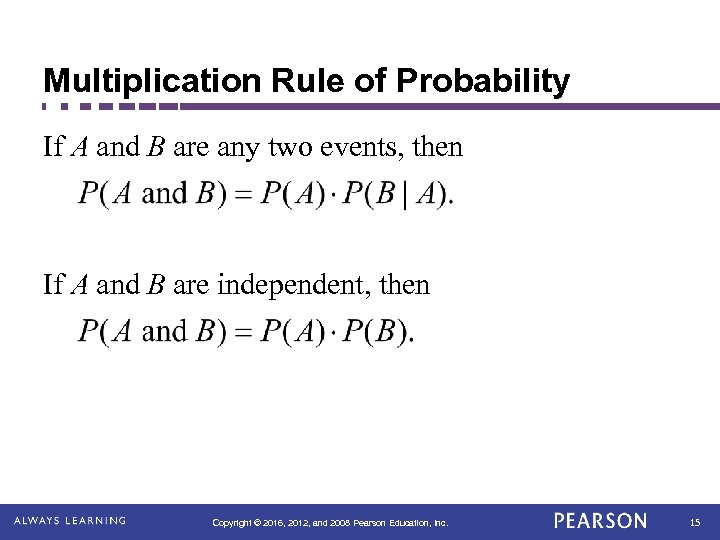Multiplication Rule of Probability If A and B are any two events, then If A and B are independent, then Copyright © 2016, 2012, and 2008 Pearson Education, Inc. 15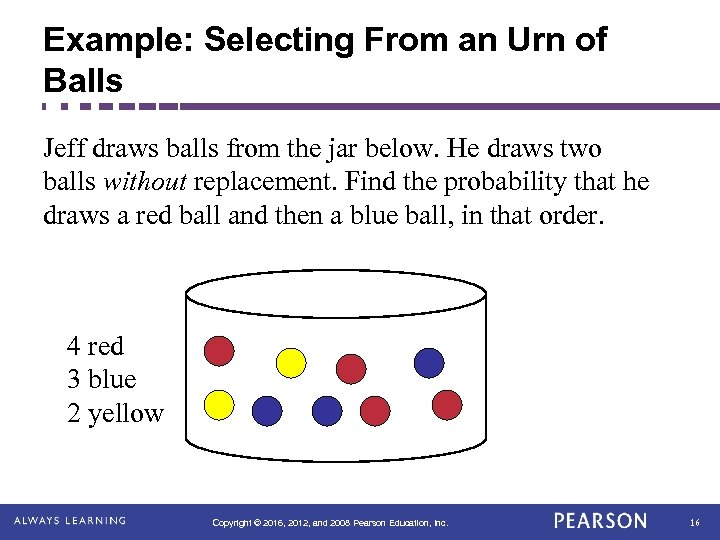Example: Selecting From an Urn of Balls Jeff draws balls from the jar below. He draws two balls without replacement. Find the probability that he draws a red ball and then a blue ball, in that order. 4 red 3 blue 2 yellow Copyright © 2016, 2012, and 2008 Pearson Education, Inc. 16Example: Selecting From an Urn of Balls JAR 4 red 3 blue 2 yellow Solution Copyright © 2016, 2012, and 2008 Pearson Education, Inc. 17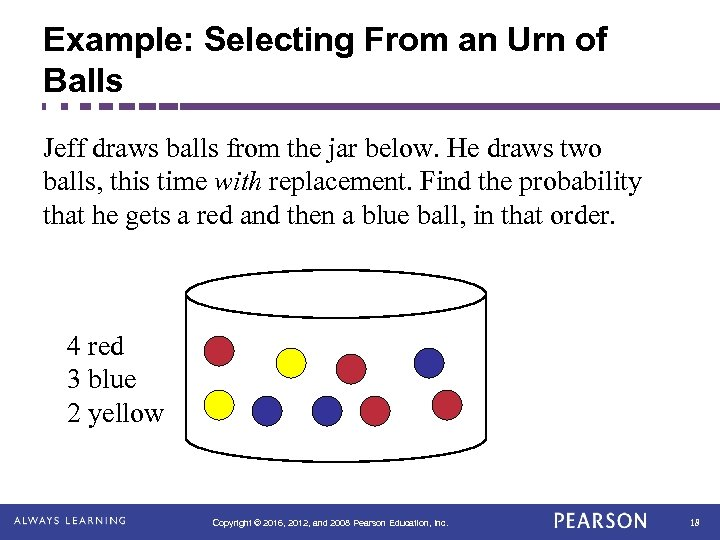Example: Selecting From an Urn of Balls Jeff draws balls from the jar below. He draws two balls, this time with replacement. Find the probability that he gets a red and then a blue ball, in that order. 4 red 3 blue 2 yellow Copyright © 2016, 2012, and 2008 Pearson Education, Inc. 18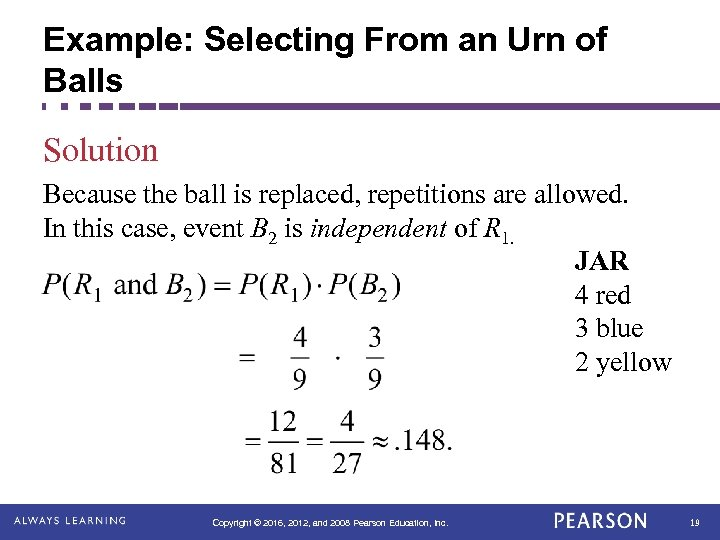Example: Selecting From an Urn of Balls Solution Because the ball is replaced, repetitions are allowed. In this case, event B 2 is independent of R 1. JAR 4 red 3 blue 2 yellow Copyright © 2016, 2012, and 2008 Pearson Education, Inc. 19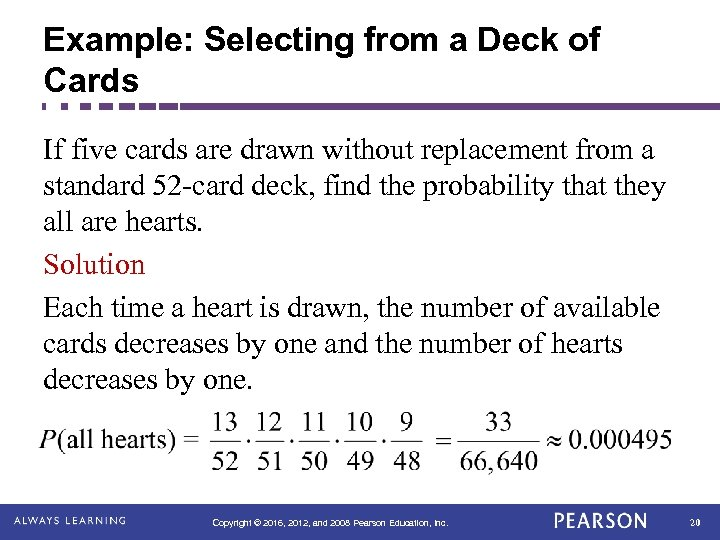Example: Selecting from a Deck of Cards If five cards are drawn without replacement from a standard 52 -card deck, find the probability that they all are hearts. Solution Each time a heart is drawn, the number of available cards decreases by one and the number of hearts decreases by one. Copyright © 2016, 2012, and 2008 Pearson Education, Inc. 20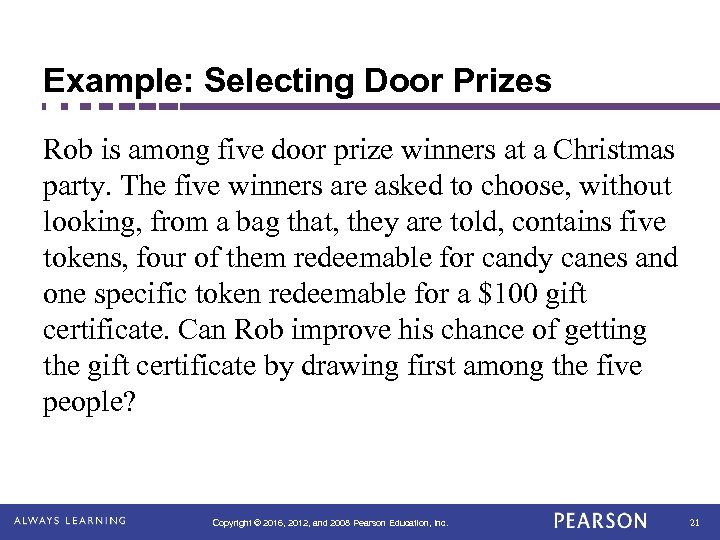Example: Selecting Door Prizes Rob is among five door prize winners at a Christmas party. The five winners are asked to choose, without looking, from a bag that, they are told, contains five tokens, four of them redeemable for candy canes and one specific token redeemable for a \$100 gift certificate. Can Rob improve his chance of getting the gift certificate by drawing first among the five people? Copyright © 2016, 2012, and 2008 Pearson Education, Inc. 21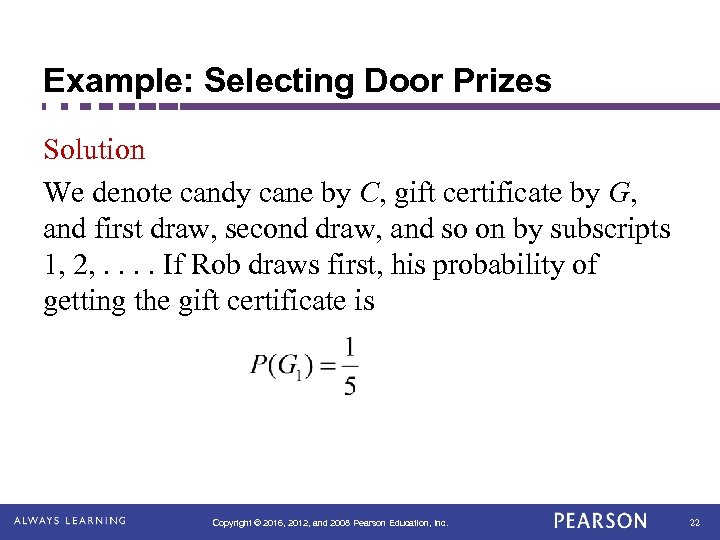Example: Selecting Door Prizes Solution We denote candy cane by C, gift certificate by G, and first draw, second draw, and so on by subscripts 1, 2, . . If Rob draws first, his probability of getting the gift certificate is Copyright © 2016, 2012, and 2008 Pearson Education, Inc. 22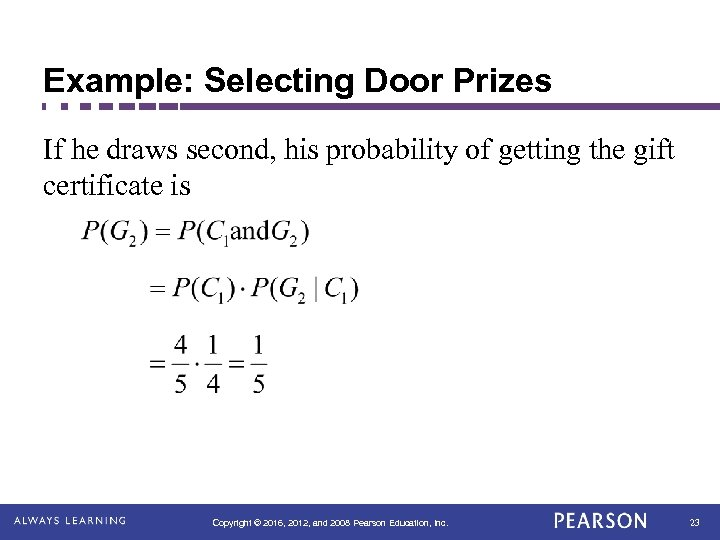Example: Selecting Door Prizes If he draws second, his probability of getting the gift certificate is Copyright © 2016, 2012, and 2008 Pearson Education, Inc. 23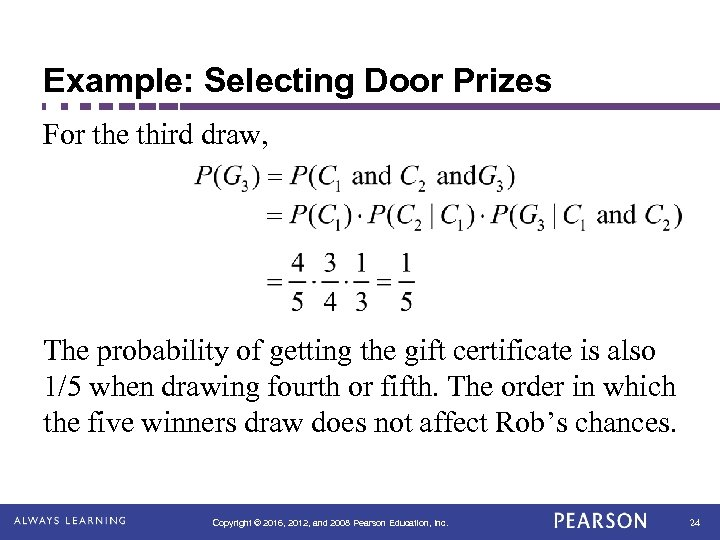Example: Selecting Door Prizes For the third draw, The probability of getting the gift certificate is also 1/5 when drawing fourth or fifth. The order in which the five winners draw does not affect Rob’s chances. Copyright © 2016, 2012, and 2008 Pearson Education, Inc. 24